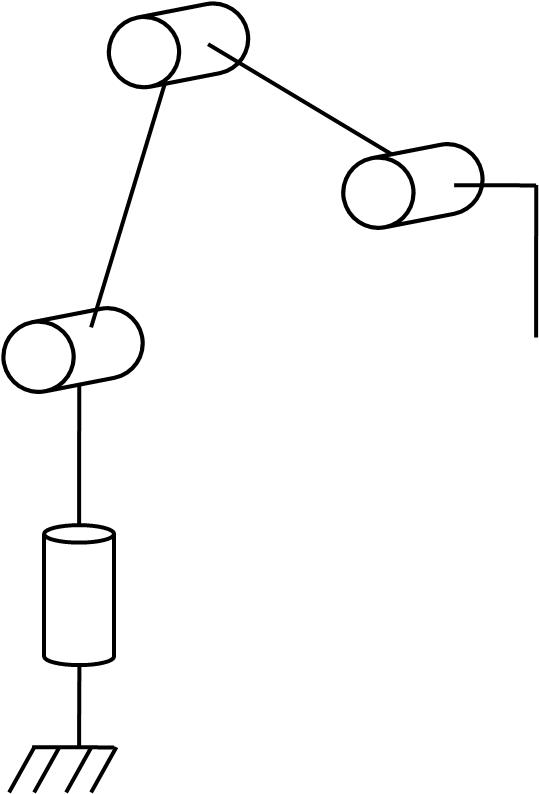Select Page
Tags: , , ,

A good exercise for programmation is to make a robotic arm reproduce a trajectory, such as drawing on a piece of paper. Once you have this knowledge, a robotic arm can be programmed to do anything you want.

# Material

• Robotic arm Handy
• 4x Servomotor SMS1012
• Servodriver
• 5V power supply
• USB cable
• Arduino UNO

# Objectif

Obj: Make a robot draw

To realize this project, we need to:

• Mount the robotic arm (Structure)
• Link the electronics to the motor and power to allow the control of the arm (Hardware)
• Write the program to make the robot follow a trajectory (Software)

# Structure

Obj: The structure should be able to carry a pen in three dimensions of space.

We used a desktop robotic arm Handy for this project. The parts are optimized for a tiny servomotor and were printed from base to wrist. An aditional pen holder was designed and printed.
that you can download here. The arm is mounted with 4 micro servomotors and the base is attached to a wooden plate.# Hardware

Obj: The board should be linked to servomotor and power supply in order to control the servomotor with a PWM signal.

• arduino UNO

Arduino board was picked, they are easy to use and there is huge community to ask for help. As the program ask for a bit of memory we chose a Arduino UNO.

• Servo driver

The arduino cannot power so much servomotors. A driver need to be add to distribute the power from another source.

This tutorial was followed to make the driver.# Software

## Objectives

;
The objective of the program can be divided in smaller tasks:

• Describe the trajectory coordinates in space
• Transform the coordintates of the trajectory into the desired angles of the robot joints.
• Control the robot position
• Convert robot angles into servomotor command value
• Send command to servomotor

## Describe the trajectory coordinates in space

The trajectory chosen is a circle for its simplicity. The parametric equations are :

For the z coordinates, we take the pen height with respect to the wrist: 20mm.

We write a function that output the desired position with respect to the time step:

```void circleTraj(double R,double x,double y,double z,int i,double Te, float Xd){
Xd=x+R*cos(2*PI*Te*i);
Xd=y+R*sin(2*PI*Te*i);
Xd=z;
Xd=0;
Xd=0;
Xd=0;
}

```

## Transform the coordintates of the trajectory into the desired angles of the robot joints

The robotic arm can be described with the Denavit-Hartenberg mehtod as a robotic chain with 4 articulated links. This method is interesting to controle articulated projects with more than 3 articulations.The method defines a cartesian frame for each joint following the same logic which result in the definition of parameters that can be used to describe mathematically the movement of the links.

Definition of the parameters with respect to geometry (DH parameters)

 j Sigmaj Thetaj Dj (mm) Aj (mm) alphaj 1 0 Th1 45 0 90 2 0 Th2 0 75 0 3 0 Th3 0 75 0 4 0 Th4 4 0 -90

To compute the desired angles from the desired position we use the inverse of the jacobian matrix defined with the denavit-hartenberg parameters. To compute matrices we use MatrixMath.h library

```void computeAngle(){
double detJ;
//    compute jacobian
// avoid singularity
// invert jacobian
if(detJ>=1){
}
qu[i]=0;
for(int j=0;j<6;j++){
qu[i]+=invJ[i][j]*Xu[j];
}
}
}
```

## Convert joint angle into servomotor command

In order to convert angles to PWM command, servomotor position with respect to the joint and PWM nominal values should be known. This conversion also depends on the cartesian frames used to describe the mechanical link with DH method.

 Servo/Joint Min impulse Max impulse Min robot Max robot 1 500 2500 -PI/2 PI/2 2 500 2500 PI 0 3 600 2600 -PI/2 PI/2 4 500 2500 -PI/2 PI/2

```int jointToImp(double x,int i) {
int imp=(x - joint_min[i]) * (imp_max[i]-imp_min[i]) / (joint_max[i]-joint_min[i]) + imp_min[i];
imp=max(imp,imp_min[i]);
imp=min(imp,imp_max[i]);
return imp;
}
```

## Send command to servomotor

To drive the servomotors, we use the basic Servo.h library available in the Arduino IDE. We write a function that sends the PWM command to each servomotor according to the requested angle.

```void servoToImp(double q[nbLinks],int velocity){
for (int i=0; i<nbLinks; i++) {
servo[i].writeMicroseconds(jointToImp(q[i],i));
delay(velocity);
}
}
```

## Control the robot position

To control servomotors, desired and actual position must be compared in real time

Robot position is compute with the angle of the joints using the transformtaion matrices defined with the denavit-hartenberg method.

```        forwardKinematics(sig,a,al,d,th,q,nbLinks,X);
```

Controle is applied to compute the next desired position

```        circleTraj(20,165,0,20,k,Te,Xd);
circleTraj(20,165,0,20,k+1,Te,Xk);
for(int i=0;i<6;i++) Xu[i]=(Xk[i]-Xd[i]+gain*Te*(Xd[i]-X[i]));
```

The desired servomotor angles are computed to reache the next desired position

```        for(int i=0;i<nbLinks;i++) qN1[i]=q[i];
computeAngle();
```

PWM command is apply for every servo.

```        servoToImp(q,10);
```

# Résultat

The robot follow the trajectory and is able to draw a circle on the paper